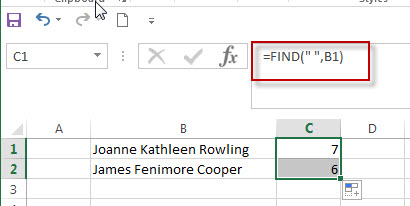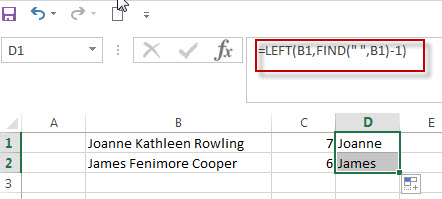# Get First Name From Full Name

This post explains how to extract the First name from full name using excel formula.

If you want to get the first name from a full name list in Column B, you can use the FIND function within LEFT function in excel. The generic formula is as follows:

`=LEFT(B1,FIND(" ",B1)-1)`

The full name is separated by the space character in Cell B1. So if you want to get the first name, you need to get the position of the first space character using the FIND function.

`=FIND(" ",B1)`The LEFT function will extract the leftmost characters based on the numeric position returned by the FIND function. Entering the below formula into the Cell B1 to get the first name from a full name in Cell B1:

`=LEFT(B1,FIND(" ",B1)-1)`